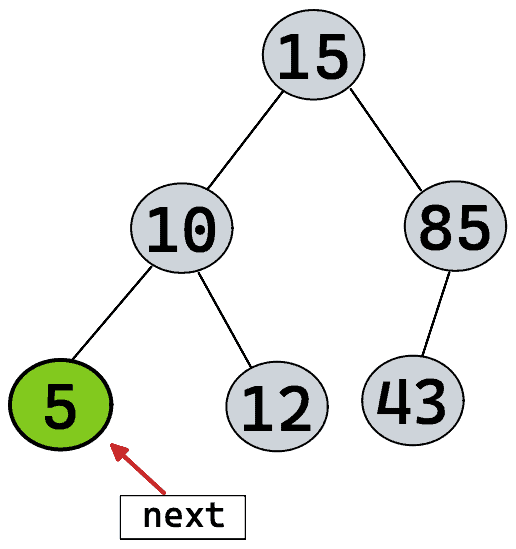# Binary Search Tree Iterator##### Problem

Implement the `BSTIterator` class that represents an iterator over the in-order traversal of a binary search tree (BST):

• `BSTIterator(TreeNode root)` Initializes an object of the `BSTIterator` class. The `root` of the BST is given as part of the constructor. The pointer should be initialized to a non-existent number smaller than any element in the BST.
• `boolean hasNext()` Returns `true` if there exists a number in the traversal to the right of the pointer, otherwise returns `false`.
• `int next()` Moves the pointer to the right, then returns the number at the pointer.

Notice that by initializing the pointer to a non-existent smallest number, the first call to `next()` will return the smallest element in the BST.

You may assume that `next()` calls will always be valid. That is, there will be at least a next number in the in-order traversal when `next()` is called.

Example 1:

```Input
["BSTIterator", "next", "next", "hasNext", "next", "hasNext", "next", "hasNext", "next", "hasNext"]
[[[7, 3, 15, null, null, 9, 20]], [], [], [], [], [], [], [], [], []]
Output
```

[null, 3, 7, true, 9, true, 15, true, 20, false]

Explanation BSTIterator bSTIterator = new BSTIterator([7, 3, 15, null, null, 9, 20]); bSTIterator.next(); // return 3 bSTIterator.next(); // return 7 bSTIterator.hasNext(); // return True bSTIterator.next(); // return 9 bSTIterator.hasNext(); // return True bSTIterator.next(); // return 15 bSTIterator.hasNext(); // return True bSTIterator.next(); // return 20 bSTIterator.hasNext(); // return False

Constraints:

• The number of nodes in the tree is in the range `[1, 105]`.
• `0 <= Node.val <= 106`
• At most `105` calls will be made to `hasNext`, and `next`.

• Could you implement `next()` and `hasNext()` to run in average `O(1)` time and use `O(h)` memory, where `h` is the height of the tree?
##### Solution

In inorder traversal, an iterator traverses to the left first, then (when there is no left child left) prints (or do something else with) the value and then traverses right. We have to mainly think about the implementation of the next() function of an iterator, as it is the most critical one.

Since the tree is already built, in the constructor of iterator we can use a stack and traverse to the left end of the tree and push all the left children in the stack. So, when someone will call next(), we can simply return the top of the stack, right? Because, when the iterator tries to find a next element, it traverses all the way back to the left of the tree and returns the left most child which we already did during the construction period and pushed all left children to the stack.

Is our job done? Not yet! We know the left-most leaf node has no children. But what about its parent node? When we will get the second next() call, we will return the second left child (from bottom). Now, this node might have a right child. So, before returning this node, we will need to check if it has a right child and push that into stack. From this point, we might have another chain of left children which we should take care of by pushing to the stack until no-one is left (same process that we did in the construction function).

This way, by using stack, we will be able to make the iterator.

###### C++ Code
```/**
* Definition for a binary tree node.
* struct TreeNode {
*     int val;
*     TreeNode *left;
*     TreeNode *right;
*     TreeNode() : val(0), left(nullptr), right(nullptr) {}
*     TreeNode(int x) : val(x), left(nullptr), right(nullptr) {}
*     TreeNode(int x, TreeNode *left, TreeNode *right) : val(x), left(left), right(right) {}
* };
*/
class BSTIterator {
public:
stack<TreeNode*> st;
BSTIterator(TreeNode* root) {
while(root != NULL){
st.push(root);
root = root->left; //we will reach until the end by traversing left, and push elements on stack. So, the top most element is the leftmoset element. When someone will call next(), it will return the top most element from stack, which is the leftmost, just like inorder traversal
}
}

int next() {
//Our iterator will return the top most stack element, which is the left most one
TreeNode* cur = st.top();
st.pop();
//we will return cur, but befor that, we need to check if it has any right child or not.
if(cur->right != NULL){
TreeNode* temp = cur->right;
while(temp != NULL){
st.push(temp);
temp = temp->left;
}
}
return cur->val;
}

bool hasNext() {
//if we have any element in stack, we have a next element for the iterator
return st.size() > 0;
}
};

/**
* Your BSTIterator object will be instantiated and called as such:
* BSTIterator* obj = new BSTIterator(root);
* int param_1 = obj->next();
* bool param_2 = obj->hasNext();
*/```
###### Complexity

Average time complexity is O(1) and space complexity is O(h) where h is the height of tree.